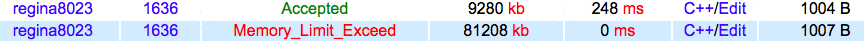# 【BZOJ 1636】 [Usaco2007 Jan]Balanced Lineup

## 1636: [Usaco2007 Jan]Balanced Lineup

Time Limit: 5 Sec   Memory Limit: 64 MB
Submit: 516   Solved: 374
[ Submit][ Status]

## Description

For the daily milking, Farmer John's N cows (1 <= N <= 50,000) always line up in the same order. One day Farmer John decides to organize a game of Ultimate Frisbee with some of the cows. To keep things simple, he will take a contiguous range of cows from the milking lineup to play the game. However, for all the cows to have fun they should not differ too much in height. Farmer John has made a list of Q (1 <= Q <= 200,000) potential groups of cows and their heights (1 <= height <= 1,000,000). For each group, he wants your help to determine the difference in height between the shortest and the tallest cow in the group.

## Input

* Line 1: Two space-separated integers, N and Q. * Lines 2..N+1: Line i+1 contains a single integer that is the height of cow i * Lines N+2..N+Q+1: Two integers A and B (1 <= A <= B <= N), representing the range of cows from A to B inclusive.

## Output

6 3 1 7 3 4 2 5 1 5 4 6 2 2

## Sample Input

* Lines 1..Q: Each line contains a single integer that is a response
to a reply and indicates the difference in height between the
tallest and shortest cow in the range.

6
3
0

## Source

RMQ水题。

#include <iostream>
#include <cmath>
#include <algorithm>
#include <cstring>
#include <cstdio>
using namespace std;
int maxx,minn,n,q,a,mi,ma;
void RMQ()
{
for (int i=1;i<=n;i++)
mi[i]=ma[i]=a[i];
for (int j=1;(1<<j)<n;j++)
for (int i=1;i+(1<<j)-1<=n;i++)
mi[i][j]=min(mi[i][j-1],mi[i+(1<<(j-1))][j-1]),
ma[i][j]=max(ma[i][j-1],ma[i+(1<<(j-1))][j-1]);
}
int Getlog(int x)
{
int now=1;
if (x==1) return 0;
for (int i=1;i<=100;i++)
{
now*=2;
if (x==now||x<now*2) return i;
}
}
void Ask(int l,int r)
{
int k=Getlog(r-l+1);
maxx=max(ma[l][k],ma[r-(1<<k)+1][k]);
minn=min(mi[l][k],mi[r-(1<<k)+1][k]);
}
int main()
{
scanf("%d%d",&n,&q);
for (int i=1;i<=n;i++)
scanf("%d",&a[i]);
RMQ();
while (q--)
{
int x,y;
scanf("%d%d",&x,&y);
Ask(x,y);
printf("%d\n",maxx-minn);
}
return 0;
}06-024308-19153
08-2245
03-05198
08-1333
06-0934
11-11272
08-13278
08-07711
10-1728
12-1947
09-27158
06-131307
05-246万+
©️2020 CSDN 皮肤主题: 技术黑板 设计师:CSDN官方博客